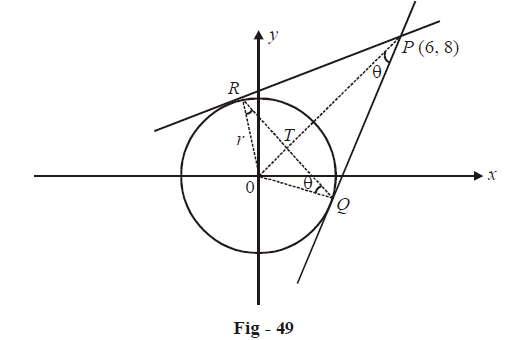# Applications of Derivatives Set 7

Go back to  'SOLVED EXAMPLES'

Example - 14

For the circle  $${x^2} + {y^2} = {r^2},$$ find the value of r for which the area a enclosed by the tangents drawn from the point P(6, 8) to the circle and the chord of contact is maximum.

Solution: From the figure drawn corresponding to the situation described in the question, observe that there will be a particular value of r for which the required area is maximum, this is  because as $$r \to 0$$ or as $$r \to 10$$ (the distance of P from the origin), the required $${\rm{area}} \to 0$$. Hence, for some value of r between 0 and 10, the area will attain its maximum value.We need to express area of $$\Delta PQR$$  in terms of some variable. As shown in the figure, assume $$\Delta OPQ = \theta .$$ Therefore$$\angle OQR = \angle OPQ = \theta .$$ From the geometry of the figure, observe how the area is obtained in the following manner

\begin{align}\qquad \quad \; &OP=10 \\ \qquad \quad \; &OT=OQ\,\sin \theta =r\,sin\theta \\ \;\;\Rightarrow \qquad &PT=\left( 10-r\,\sin \theta \right) \\ \qquad \quad & OT=r\,\cos \theta \\ \;\Rightarrow \qquad & RQ=2r\cos \theta \\ \end{align}

Also, in$$\Delta OPQ,$$

OQ = r = 10 sin$$\theta$$

The required area of $$\Delta PQR,$$

\begin{align}&\;A = \frac{1}{2} \times RQ \times PT\\\\ &\quad = \frac{1}{2} \times 2r\cos \theta \times \left( {10 - r\sin \theta } \right)\\\\&\quad = 10\sin \theta \cos \theta \times \left( {10 - 10{{\sin }^2}\theta } \right)\\\\&\quad = 100\sin \theta {\cos ^3}\theta \end{align}

We have been able to express the area A in terms of $$\theta$$.

For maximum area,

\begin{align}&\frac{{dA}}{{d\theta }} = 0\,\,\,{\rm{and}}\,\,\frac{{{d^2}A}}{{d{\theta ^2}}} < 0\\\\&\frac{{dA}}{{d\theta }} = 100\left\{ { - 3{{\cos }^2}\theta\; {{\sin }^2}\theta + {{\cos }^4}\theta } \right\}\\\\&\,\,\,\,\,\,\,\;\; = 100\;{\cos ^2}\theta \left\{ {{{\cos }^2}\theta - 3\;{{\sin }^2}\theta } \right\}\end{align}

This is 0 when  $${\cos ^2}\theta - 3{\sin ^2}\theta = 0$$

\begin{align} &\Rightarrow \quad {\tan ^2}\theta = \frac{1}{3}\\\\ &\Rightarrow\quad \tan \theta = \frac{1}{{\sqrt 3 }}\\\\ & \Rightarrow \quad \theta = \frac{\pi }{6}\end{align}

Verify that  \begin{align}{\left. {\frac{{{d^2}A}}{{d{\theta ^2}}}} \right|_{\theta = \pi /6}} < 0\end{align}

$$\Rightarrow \quad \theta = \pi /6 \text{ is a point of local maximum for A.}$$

\begin{align}& \Rightarrow \quad {r_{\max }} = 10\sin {\theta _{\max }}\\\\ &\qquad\qquad= 10\sin \pi /6\\\\ &\qquad\qquad= 5\end{align}

Example - 15

Show that the normal to \begin{align}xy = {c^2}\,\,at\,\,A\left( {{t_1},\frac{{{c^2}}}{{{t_1}}}} \right)\end{align} meets the curve again at the point\begin{align}B\left( {{t_2},\frac{{{c^2}}}{{{t_2}}}} \right){\rm{if}}\,\,t_1^3{t_2} = - {c^4}\end{align}

Solution: What we are required to do here is write the equation of the normal to the curve at A, find the other intersection point B of this normal with the curve, and show that the co-ordinates of A and B satisfy the given condition.

Differentiating the equation of the given curve, we get

\begin{align}&\qquad\;\, x\frac{{dy}}{{dx}} + y = 0\\\\ &\Rightarrow \quad \frac{{dy}}{{dx}} = \frac{{ - y}}{x}\\\\& \Rightarrow \quad {\left. {\frac{{dy}}{{dx}}} \right|_{\left( {{t_1},\frac{{c_1^2}}{{{t_1}}}} \right)}} = \frac{{ - {c^2}}}{{t_1^2}}\end{align}

Equation of normal at A:

$y - \frac{{{c^2}}}{{{t_1}}} = \frac{{t_1^2}}{{{c^2}}}\left( {x - {t_1}} \right)$

Since this normal meets the curve again at B, the assumed co-ordinates of B must satisfy the equation of this normal.

\begin{align}& \Rightarrow \quad \frac{{{c^2}}}{{{t_2}}} - \frac{{{c^2}}}{{{t_1}}} = \frac{{t_1^2}}{{{c^2}}}\left( {{t_2} - {t_1}} \right)\\\\ &\Rightarrow \quad {c^2}\frac{{\left( {{t_1} - {t_2}} \right)}}{{{t_1}{t_2}}} = \frac{{t_1^2}}{{{c^2}}}\left( {{t_2} - {t_1}} \right)\\\\& \Rightarrow \quad t_1^3{t_2} = - {c^4}\end{align}

Learn from the best math teachers and top your exams

• Live one on one classroom and doubt clearing
• Practice worksheets in and after class for conceptual clarity
• Personalized curriculum to keep up with school